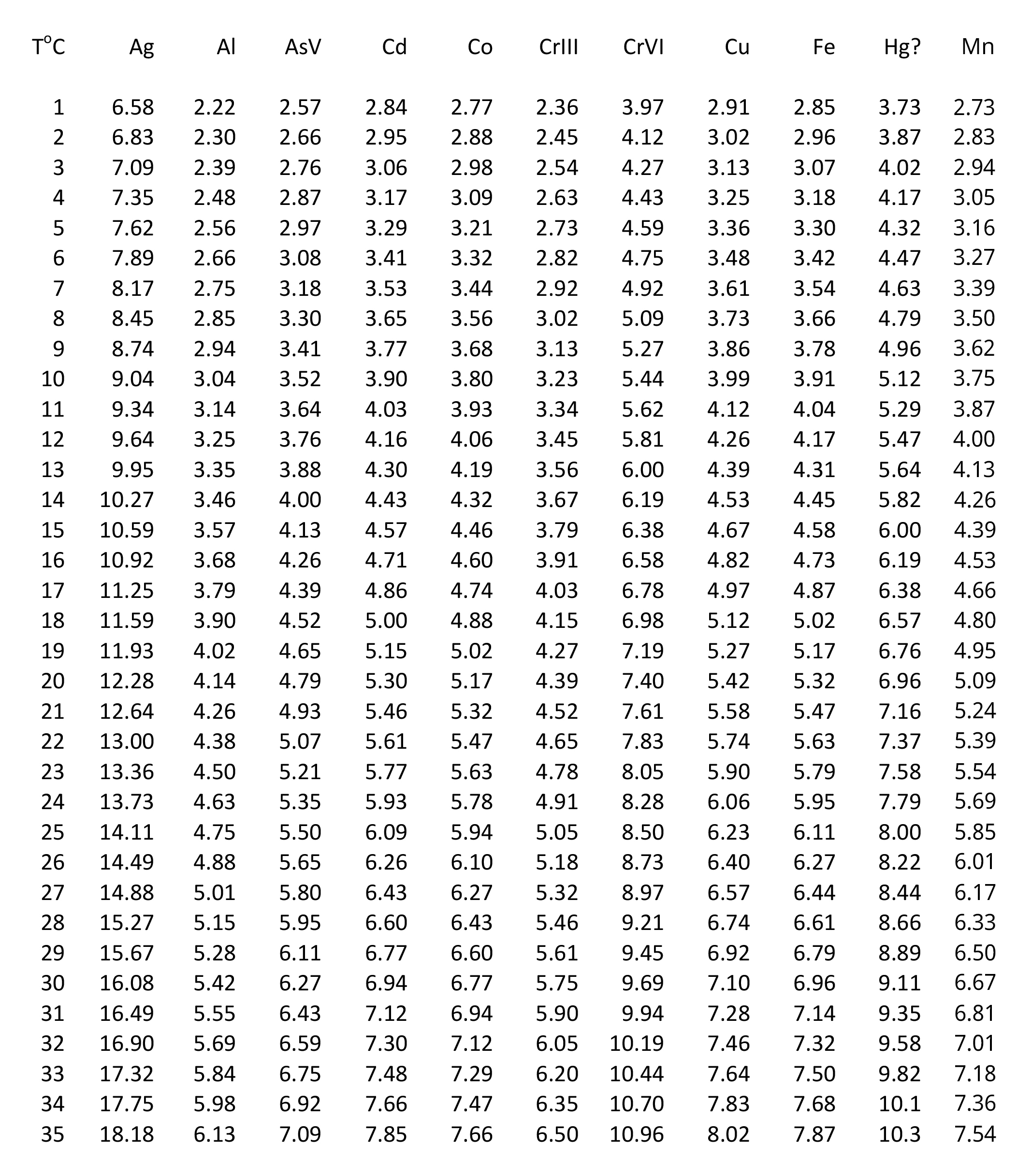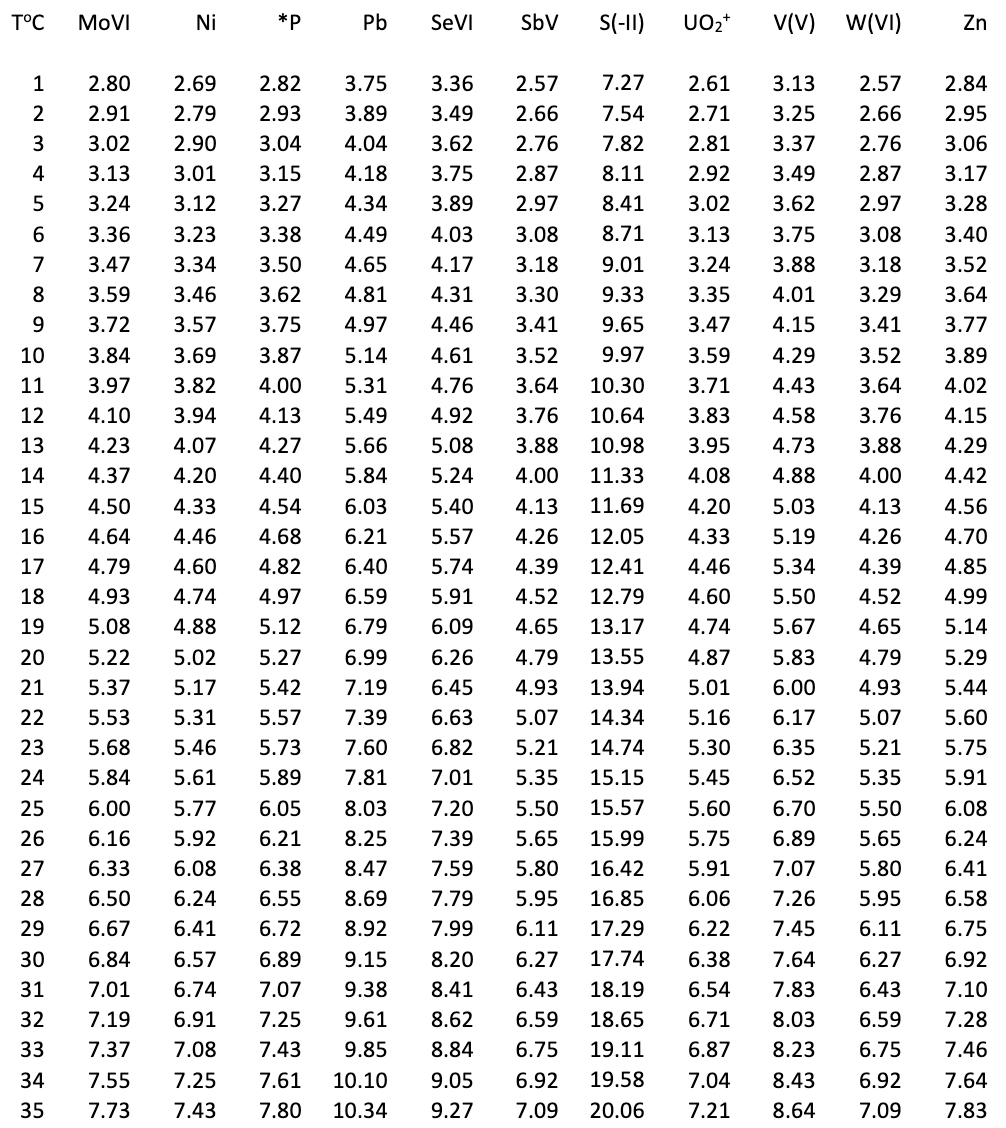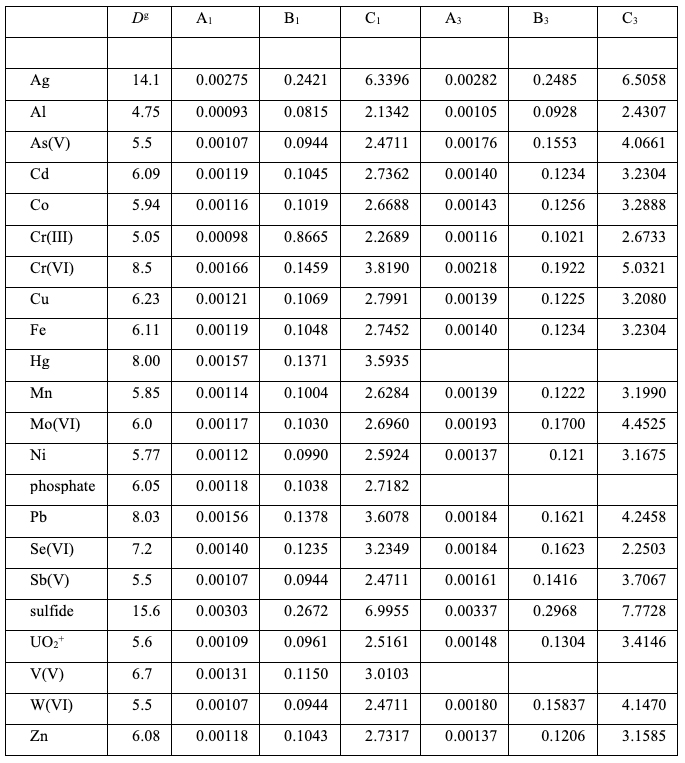# Diffusion Coefficients

/Diffusion Coefficients
Diffusion Coefficients 2019-11-25T13:00:54+00:00

Diffusion Coefficients in the standard DGT gel (agarose crosslinked polyacrylamide, APA) from 1 to 35°C (calculated using the equations provided below). Multiply the number by 10-6 to give D in cm2 s-1.There is no agreement on the diffusion coefficients for mercury. These are estimated values based on a critical assessment of the information provided in Diffusive Gradients in Thin-films (DGT) for Environmental Measurements, Editor: William Davison, Cambridge University Press, 2016.

Diffusion Coefficients in an agarose crosslinked polyacrylamide (APA) gel from 1 to 35°C (calculated using the equations provided below).*P here refers to phosphate. S(-II) refers to sulfide. The data are based on 90% of the diffusion coefficient of HS- in water at 25°C.

Table for calculating diffusion coefficients of commonly measured analytes in the APA gel and water at any temperature between 1 and 35°C.

The temperature dependence of the diffusion coefficient, D, of each analyte is expressed as a second order polynomial (quadratic) of the form D = AT2 + BT + C where T is the temperature in oC. The number obtained from the equation should be multiplied by 10-6 to obtain a value of D in cm2 s-1. Substitution of the coefficients A1, B1 and C1 into the above equation provides values of diffusion coefficients of each analyte in an APA gel, with the value at 25oC shown in column 2. Substitution of the coefficients A3, B3 and C3 into the above equation provides values of diffusion coefficients of each analyte in water. All data except mercury and sulfide are adapted from the Appendix of Diffusive Gradients in Thin-films (DGT) for Environmental Measurements, Editor: William Davison, Cambridge University Press, 2016. The sources of the data and assumptions made in their derivation are provided in the book. Sulfide in the gel is based on 90% of the value of HS in water at 25oC. Values for Hg are for an agarose gel and are as yet unvalidated.Diffusion coefficients for organic substances. As new methods for measuring organics using DGT are developed, diffusion coefficients are being determined, as provided in information for organic analytes. While theses values are expected to be reliable, it is advisable to recognise that generally there are as yet insufficient data to establish them as consensus values.